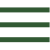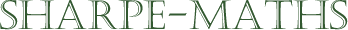Previous topic: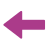Current topic:
Pythagoras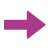Next topic:
Trigonometry (introduction)

### Pythagoras' Theorem

This is one of the most extraordinary theorems in all of mathematics. Discovered in the 6th century BCE, in a small, rather cultish community in southern Italy, this theorem gives us the connection between the lengths of the three sides of any right triangle.1 It is considered unlikely that Pythagoras himself discovered the theorem, though one of the members of the commune did so and according to legend, was immediately chucked off a cliff to his death, due to the fact that the theorem means that it is impossible to measure accurately the hypotenuse of most right triangles. The Pythagoreans has some fairly bizarre ideas, including a prohibition on beans of any sort. Eating them was a complete no-no and even walking through a field of fava beans was against the rules.2

The Pythagoreans were also credited with making major discoveries about music, though as we have no records of any of their writings, these things are mostly hearsay. It is, however, reasonably sure that they were class A nutters, and mathematics has been littered with class A nutters throughout the ages, which is fun, because nutters are much more interesting than non-nutters.3 They were also heavily into Numerology so would have fitted in very well in some areas of Twitter and Quora.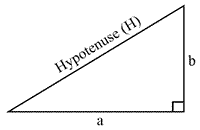Let's now look at the mathematics. Firstly, we should define our terms:

• The hypotenuse is the longest side of a right triangle.
• It can also be thought of as the side opposite the right angle, by which we mean that the hypotenuse is the only side which does not touch the right angle.
• We can also say that $a$ and $b$ are adjacent to the right angle, where adjacent means "next to".

Pythagoras' Theorem states that

In any right triangle the square of the hypotenuse is equal to the sum of the squares on the other two sides.
$a^2+b^2=H^2$   where $H$ is the length of the hypotenuse, and $a$ and $b$ are the lengths of the other two sides.

In this section, we will learn to find

1. the length of a hypotenuse when we know the other two sides.
2. the length of an unknown side, when we know the lengths of the hypotenuse and also that of the other side.

Now have a look at the worked examples, where we will go into this process in more detail.

1. Right triangles are often called right angled triangles as well, though I will be using the more succinct form right triangles as it is quicker to type. When you see the word right in mathematics, it usually means upright or at right angles. Perpendicular also means at right angles to. We sometimes also use the word orthogonal but generally only in higher level mathematics and when the author is trying to obfuscate (use inaccessible language in order to restrict the readership).

2. Whether there was also a prohibition against "a nice Chianti", legend does not tell.

3. When I use the word "nutter", I am not referring to a mental illness of any sort. I am simply saying that they were a bit bonkers!